• 基于离散傅里叶变换测量矩阵的计算重​​影成像算法研究研究论文
• 基于并行光学向量矩阵乘法器原理的离散傅里叶变换方法，利用光传播的高速和低损耗特点，提出以相位空间光调制器为核心变换矩阵的全光并行离散傅里叶变换方法，并通过实验进行了验证。实验结果显示，所提出的全光并行...
• 首先，不难将DFT写成矩阵形式，回忆之前的推导，DFT表达式为: Ff––––=∑n=0N−1f[n]––––ω––−nFf_=∑n=0N−1f[n]_ω_−n\underline{{\scr F}f} = \sum_{n=0}^{N-1} \underline{f[n]} \underline{\...
本文主要罗列一下DFT的相关公式

首先，不难将DFT写成矩阵形式，回忆之前的推导，DFT表达式为:
$\underline{\mathscr{F}f} = \sum_{n=0}^{N-1} \underline{f[n]} \underline{\omega}^{-n}$
写成矩阵形式就是：
$\large\begin{bmatrix}\underline{\mathscr{F}f}\\\underline{\mathscr{F}f}\\\vdots\\\underline{\mathscr{F}f[N-1]}\end{bmatrix} = \begin{bmatrix}1 & 1 & \cdots & 1 \\1 & \underline{\omega}^{-1 \cdot 1} & \cdots & \underline{\omega}^{- 1 \cdot (N-1)} \\\vdots & \vdots & \ddots & \vdots \\1 & \underline{\omega}^{-(N-1) \cdot 1} & \cdots & \underline{\omega}^{-(N-1)^2}\end{bmatrix}\begin{bmatrix}\underline{f } \\\underline{f} \\\vdots\\\underline{f[N-1]} \end{bmatrix}$
我们发现，DFT其实是一个对称矩阵，即
$\huge\underline\mathscr{F}^T = \underline\mathscr{F}\tag1$
根据上一章中的运算，还可以知道：
$\huge\underline\mathscr{F}^{-1} \underline\mathscr{F}= \underline\mathscr{F} \underline\mathscr{F}^{-1} = NI\tag2$
结合$(1)$，$(2)$两式，有：
$\huge\underline\mathscr{F}^* \underline\mathscr{F}= \underline\mathscr{F} \underline\mathscr{F}^* = NI$

DFT的对偶性：

$\huge\underline{f}^- [n] = \underline{f}[-n]$

$\huge\underline\mathscr{F}\left( \underline{f}^- \right) = \left(\underline{\mathscr{F} f} \right)^-$

$\huge\underline{\mathscr{F}\mathscr{F}f} = N f^-$

$(3)$ 也是与连续傅里叶变换情况不同的地方

类似连续情况，离散情况下的两个常用信号
a. $\underline{1} = (1, 1, \dots, 1)$ 表示各处均为1的离散信号
b. $\underline{\delta_k} = (0, \dots, 0, 1, 0, \dots, 0)$ 表示 $k$ 处为 $1$，其余均为 $0$ 的离散信号
接下来我们来推导几个常用的DFT公式

$\underline{\mathscr{F}\delta_k}$

\begin{aligned} \underline{\mathscr{F}\delta_k} &= \sum_{n=0}^{N-1} \underline{\delta_k [n]} \underline{\omega}^{-n}\\ &= 1\cdot \underline{\omega}^{-k}\\ &= \underline{\omega}^{-k} \end{aligned}
特别地，$\underline{\mathscr{F}\delta}=\underline{1}$

$\underline{\mathscr{F}\omega^k}$

$\underline{\mathscr{F}\omega^k} = \sum_{n=0}^{N-1} \underline{\omega [n]}^k \underline{\omega}^{-n}$
我们看它的第$m$项，
\begin{aligned} \underline{\mathscr{F}\omega[m]}^k &= \sum_{n=0}^{N-1} \underline{\omega [n]}^k \underline{\omega[m]}^{-n}\\ &= \sum_{n=0}^{N-1} e^{2\pi i\frac{nk}{N}} e^{-2\pi i\frac{mn}{N}}\\ &= \sum_{n=0}^{N-1} e^{2\pi i\frac{n(k-m)}{N}}\\ &= \begin{cases} 0 & k \neq m\\ N & k=m \end{cases} \end{aligned}
也就是说 $\underline{\mathscr{F}\omega^k}$ 只有在 $m=k$ 的时候值为 $N$，其他地方值均为 $0$，因此
$\underline{\mathscr{F}\omega^k} = N \underline{\delta^k}$


展开全文• 目录傅里叶变换一维连续傅里叶变换一维离散傅里叶变换傅里叶矩阵Matlab中函数的含义 傅里叶变换 傅立叶变换，表示能将满足一定条件的某个函数表示成三角函数（正弦和/或余弦函数）或者它们的积分的线性组合。在...
目录傅里叶变换一维连续傅里叶变换一维离散傅里叶变换傅里叶矩阵Matlab中fft函数和ifft的含义

傅里叶变换

傅立叶变换，表示能将满足一定条件的某个函数表示成三角函数（正弦和/或余弦函数）或者它们的积分的线性组合。在不同的研究领域，傅立叶变换具有多种不同的变体形式，如连续傅立叶变换和离散傅立叶变换。

一维连续傅里叶变换

傅里叶变换
$F(\omega)=\mathcal{F}[f(t)]=\int_{-\infty}^{+\infty}f(t)e^{-i\omega t}dt \quad\tag{1}$
傅里叶逆变换
$f(t)=\mathcal{F}^{-1}[F(\omega)]=\dfrac{1}{2\pi} \int_{-\infty}^{+\infty}F(\omega) e^{i \omega t} d\omega \quad\tag{2}$

其中由欧拉公式 $e^x=\cos x +i \sin x$ 有 $e^{-i\omega t}=\cos \omega t -i \sin \omega t$ .

这样，把$e^{-i\omega t}=\cos \omega t -i \sin \omega t$ 代入傅里叶变换右端，直观地看，该公式实际上就是把左边关于$\omega$的函数$F(\omega)$用右边（无穷项）三角函数的线性组合来表示。（积分本质是求和）。
可以注意到的一点是，右端项的函数$f$的自变量是$t$,左端项函数$F$的自变量是$\omega$,实际上这两个字母在通信领域有自己的含义：$t$ 代表时间，$\omega$ 代表$角频率$，所以说傅里叶变换的核心是从时域到频域的变换，而这种变换是通过一组特殊的正交基（三角函数正交基）来实现的。

为什么要有这样的变换?

三角函数正交基（也称为三角函数正交系）：
$\{1,\cos x,\sin x,\cos 2x,\sin 2x,\dots \cos nx,\sin nx\}$
三角函数系中，任何两个不相同的函数的乘积在$[-\pi,\pi]$上的积分都是零，即：
$\int_{-\pi}^{\pi}\cos nx dx=\int_{-\pi}^{\pi}\sin nx dx=0,\\ \int_{-\pi}^{\pi}\cos mx\cos nx dx=0\quad (m \neq n)\\ \int_{-\pi}^{\pi}\sin mx\sin nx dx=0\quad (m \neq n)\\ \int_{-\pi}^{\pi}\cos mx\sin nx dx=0\quad$
而任何一个函数的平方在$[-\pi,\pi]$上的积分都不等于零，即：
$\int_{-\pi}^{\pi}\cos^2 nx dx=\int_{-\pi}^{\pi}\sin^2 nx dx=\pi,\\ \int_{-\pi}^{\pi}1^2 dx=2\pi.$

正交函数系：若两个函数 $\varphi$ 和 $\psi$ 在$[a,b]$ 上可积，且 $\int_a^b \varphi(x)\psi(x)dx=0,$ 则称函数$\varphi$ 和 $\psi$ 在$[a,b]$ 上是正交的。

$\quad$因此三角函数系是正交函数系。
一维离散傅里叶变换
其实函数可以被理解为无限维的向量（这里考虑有限向量），只是需要把它们的定义域理解成自然数集合$\{0,1 ,2 ,3\dots\}$。所以上上面的连续傅里叶变换公式实际上也可以被离散称为向量的形式，关键在于怎么把连续的自变量离散成有限的自然数点集。
现在我们用向量 $x=\{x(k)\}_{k=0}^{N-1}$ 代表被离散的函数 $f$ ， $y=\{y(n)\}_{n=0}^{N-1}$ 代表被离散的函数 $F$。
再次将傅里叶变换公式抄下来：
$F(\omega)=\mathcal{F}[f(t)]=\int_{-\infty}^{+\infty}f(t)e^{-i\omega t}dt$

注意到需要被离散的自变量有 $t$ 和 $\omega$ :
$t:\{0,1,2,\dots,N-1\}$;
$\omega:\{0,\dfrac{2 \pi}{N},2 \dot \dfrac{2 \pi}{N},\dots,(N-1)\dfrac{2 \pi}{N}\}$

所以：

一维离散傅里叶公式：
$y(k)=\sum_{n=o}^{N-1}x(n)(e^{-\frac{2 \pi}{N}i})^{kn},\quad k=0,1,\dots N-1\quad\tag{3}$
一维离散逆傅里叶公式：
$x(n)=\dfrac{1}{n}\sum_{k=o}^{N-1}y(k)(e^{\frac{2 \pi}{N}i})^{kn},\quad n=0,1,\dots N-1 \quad\tag{4}$

将一维离散傅里叶公式展开成矩阵向量相乘的形式：
$\left( \begin{matrix} y(0)\\ y(1)\\ \vdots \\y(N-1) \end{matrix} \right)= \left( \begin{matrix} w^{-0\cdot0}& w^{-0\cdot1} &\cdots & w^{-0\cdot(N-1)}\\ w^{-1\cdot1}& w^{-1\cdot1} &\cdots & w^{-1\cdot(N-1)}\\ \vdots&\vdots&\ddots & \vdots\\ w^{-(N-1)\cdot1}& w^{-(N-1)\cdot1} &\cdots & w^{-(N-1)\cdot(N-1)} \end{matrix} \right) \left( \begin{matrix} x(0)\\ x(1)\\ \vdots \\x(N-1) \end{matrix} \right) \quad(5)$

其中 $w=e^{\frac{2 \pi}{N}i}$.

傅里叶矩阵
其实$(5)$式中右端矩阵中的 $w$ 是复数n阶单位根（$n^{th}$ roots of unity）:
$\{1,w,w^2,\cdots,w^{n-1}\}$

说明：$n$为 给定正实数
$w=e^{\frac{2 \pi}{n}i}=\cos \dfrac{2\pi}{n}+i \sin \dfrac{2\pi}{n}$.
解释：复数n阶单位根是方程 $z^n=1$ 的所有解。
性质：
a.)   $w^n=1$  ,
b.)   $w\bar{w}=1$,
c.)   $\bar{w}=w^{-1}$,
d.)   $\bar{w}^k=w^{-k}=w^{n-k}$,
e.)   $1+w+w^2+\cdots+w^{n-1}=0.$

我们用$F(=F_n)$来表示$n$阶傅里叶矩阵，则：
$F^*=\dfrac{1}{\sqrt{n}}\{w^{(i-1)(j-1)}\}=\dfrac{1}{\sqrt{n}}\left( \begin{matrix} 1&1&1&\cdots&1\\ 1&w&w^2&\cdots&w^{(n-1)}\\ 1&w^2&w^4&\cdots&w^{2(n-1)}\\ \vdots&\vdots&\vdots&\ddots&\vdots\\ 1&w^{n-1}&w^{2(n-1)}&\cdots&w^{(n-1)(n-1)} \end{matrix} \right) \quad\tag{6}$
根据上面所述的复数n阶单位根的性质我们知道序列 $w^k,k=0,1,\cdots$是周期序列，因此实际上矩阵$F$中只有$n$个不同的元素，即：
$F^*=\dfrac{1}{\sqrt{n}}\left( \begin{matrix} 1&1&1&\cdots&1\\ 1&w&w^2&\cdots&w^{(n-1)}\\ 1&w^2&w^4&\cdots&w^{(n-2)}\\ \vdots&\vdots&\vdots&\ddots&\vdots\\ 1&w^{n-1}&w^{(n-2)}&\cdots&w \end{matrix} \right) \quad\tag{7}$

注意 * 代表共轭对称
$F^*$矩阵的放缩系数 $\dfrac{1}{\sqrt{n}}$ 是为了保证$F^*$的每一列都是单位向量（长度为1）.
$F$和$F^*$都是对称矩阵：$F=F^{T} ,\quad F^*=(F^*)^T=\bar{F},\quad F=\bar{F}^*.$
$F$是酉矩阵：$FF^*=F^*F=I \quad or\quad F^{-1}=F^*$.

这时候回头看$(5)$式会发现右端的 $N \times N$ 矩阵实际上就是 $\sqrt{N}\cdot F^*$
Matlab中fft函数和ifft的含义
具体参看：官方文档_fft
这里以最简单的一种用法为例，$x$ 和 $y$ 表示两个 $n$ 维向量：

y=fft(x)=$\sqrt{N}\cdot F^*x$
x=ifft(y)=$\dfrac{1}{\sqrt{N}}\cdot Fy$


展开全文算法 线性代数 信号处理
• 计算以下图像的离散傅里叶变换 f= f=\begin{bmatrix}1&4&4&1\\2&4&4&2\\2&4&4&2\\1&4&4&1\end{bmatrix} f=⎣⎢⎢⎡​1221​4444​4444​1221​...
例题
计算以下图像的离散傅里叶变换
$f=\begin{bmatrix}1&4&4&1\\2&4&4&2\\2&4&4&2\\1&4&4&1\end{bmatrix}$
计算方式当然不是通过原始公式硬算，而是构造权值矩阵来计算。
考虑二维DFT的分解：
$F(u,v)=\sum^{M-1}_{x=0}\sum^{N-1}_{y=0}f(x,y)e^{-j2\pi (ux/M+vy/N)}\\=\sum^{M-1}_{x=0}e^{-j2\pi ux/M}\sum^{N-1}_{y=0}f(x,y)e^{-j2\pi vy/N}\\=\sum^{M-1}_{x=0}e^{-j2\pi ux/M}F(x,v)$
注意到，对每一个$x$，都有：
$F(x,v)=\sum^{N-1}_{y=0}f(x,y)e^{-j2\pi vy/N}$
因此，当$N=4$时：
$F(x,0)=\sum^{N-1}_{y=0}f(x,y)\\F(x,1)=\sum^{N-1}_{y=0}f(x,y)e^{-j\pi y/2}\\F(x,2)=\sum^{N-1}_{y=0}f(x,y)e^{-j\pi y}\\F(x,3)=\sum^{N-1}_{y=0}f(x,y)e^{-j3\pi y/2}$
固定$x$时，每个$F(x,v)$可视为$f(x,y)$这个行向量与相应的权值向量（列向量）的内积，如$F(x,1)$的公式中对应的权值向量就是$\begin{bmatrix}1&-j&-1&j\end{bmatrix}$。
因此构造权值矩阵$A_y$：
$A_y=\begin{bmatrix}1&1&1&1\\1&-j&-1&j\\1&-1&1&-1\\1&j&-1&-j\end{bmatrix}$
同理可构造权值矩阵$A_x=A_y$，因此傅里叶变换为：
$F=A_xfA_y=\begin{bmatrix}1&1&1&1\\1&-j&-1&j\\1&-1&1&-1\\1&j&-1&-j\end{bmatrix}\begin{bmatrix}1&4&4&1\\2&4&4&2\\2&4&4&2\\1&4&4&1\end{bmatrix}\begin{bmatrix}1&1&1&1\\1&-j&-1&j\\1&-1&1&-1\\1&j&-1&-j\end{bmatrix}\\=\begin{bmatrix}44&-10-10j&0&-10+10j\\-2-2j&-2j&0&-2\\0&0&0&0\\-2+2j&-2&0&2j\end{bmatrix}$
注意这两个权值矩阵对所有4x4的图像都适用，可通过matlab fft2函数检验
傅里叶反变换的权值矩阵为：
$\frac{1}{16}*\begin{bmatrix}1&1&1&1\\1&j&-1&-j\\1&-1&1&-1\\1&-j&-1&j\end{bmatrix}$
类似地，构造3x3图像的权值矩阵：
$A_x=A_y=\begin{bmatrix}1&1&1\\1&-1/2-\sqrt{3}/2j&-1/2+\sqrt{3}/2j\\1&-1/2+\sqrt{3}/2j&-1/2-\sqrt{3}/2j\end{bmatrix}$


展开全文• 离散傅里叶变换（DFT）–有限长序列的离散频域表示 离散傅里叶变换（DFT）是数字信号处理中非常有用的一种变换，因为它是频域也离散化的一种傅里叶变换。也就是说，时域和频域都离散化了，这样使计算机对信号的时域...
文章目录一、离散傅里叶变换（DFT）--有限长序列的离散频域表示1、主值区间、主值序列2、DFT的定义2.1、正变换：2.2、反变换：3、DFT用矩阵表示4、DFT与DFS的关系5、DFT与DTFT、Z变换的关系 -- 频域抽样6、离散傅里叶变换对x(n)与X(k)中各参量间的关系7、DFT 隐含的周期性二、DFT的主要性质1、线性2、圆周移位性质2.1、圆周移位序列2.2、圆周移位性质2、圆周共轭对称性质2.1、圆周对称中心
一、离散傅里叶变换（DFT）–有限长序列的离散频域表示
离散傅里叶变换（DFT）是数字信号处理中非常有用的一种变换，因为它是频域也离散化的一种傅里叶变换。也就是说，时域和频域都离散化了，这样使计算机对信号的时域、频域都能进行计算；另外DFT作为有限长序列的一种傅里叶表示法在理论上相当重要；最后由于DFT有多种快速算法。使得信号处理速度有非常大的提高。
$\color{red}{离散傅里叶变换（DFT）是离散傅里叶级数（DFS）引申出来的， }$ 这二者的时域、频域都是离散的，因而它们的时域、频域又必然是周期的。但是 DFT 是针对有限长序列的，实际上，它是取周期序列的 DFS 的一个周期的对应关系来加以定义和研究的，因而它隐含有周期性的。
1、主值区间、主值序列
设 x(n) 为有限长序列，只在 0≤n≤N-1 处有值，可以把它看成是以 N 为周期的周期性序列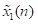的第一个周期（0≤n≤N-1），这第一个周期［0，N-1］就称为主值区间，主值区间的序列 x(n) 就称为主值序列，则有：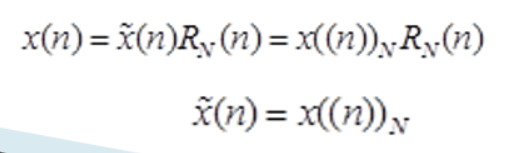2、DFT的定义
设 x(n) 为M点有限长序列，即在 0≤n≤M-1 内有值，则可定义 x(n) 的 N 点（N≥M。当N＞M时，补N-M个零值点）离散傅里叶变换定义为：
2.1、正变换：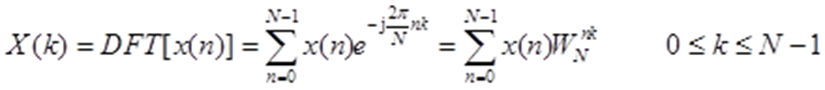2.2、反变换：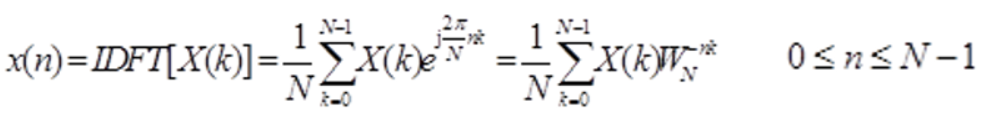3、DFT用矩阵表示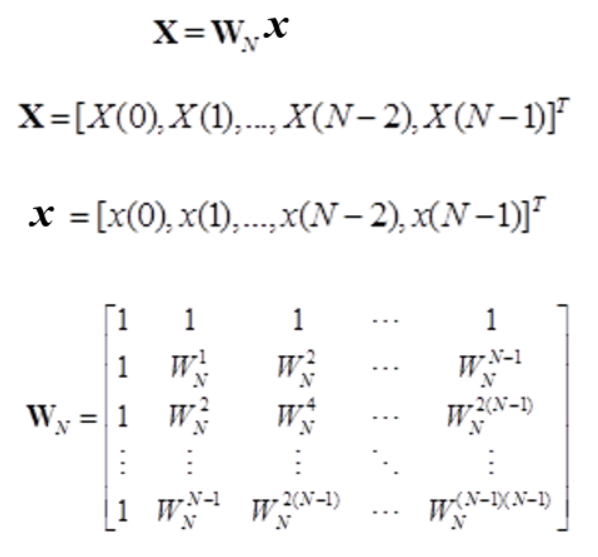IDFT也可用矩阵表示：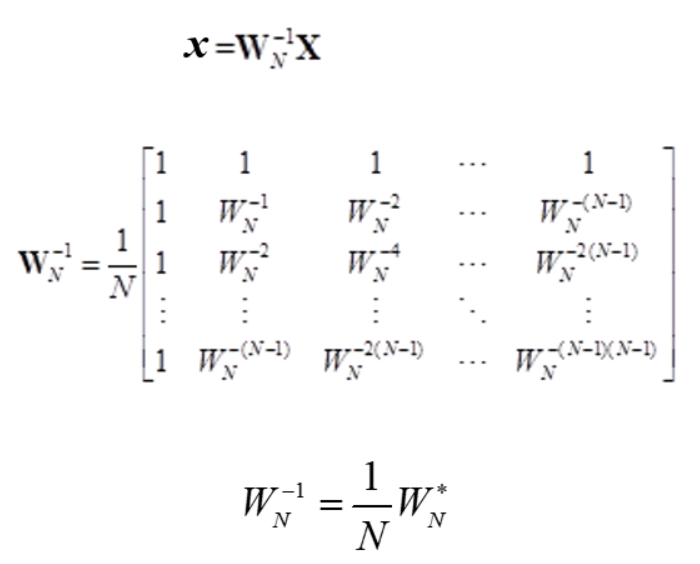4、DFT与DFS的关系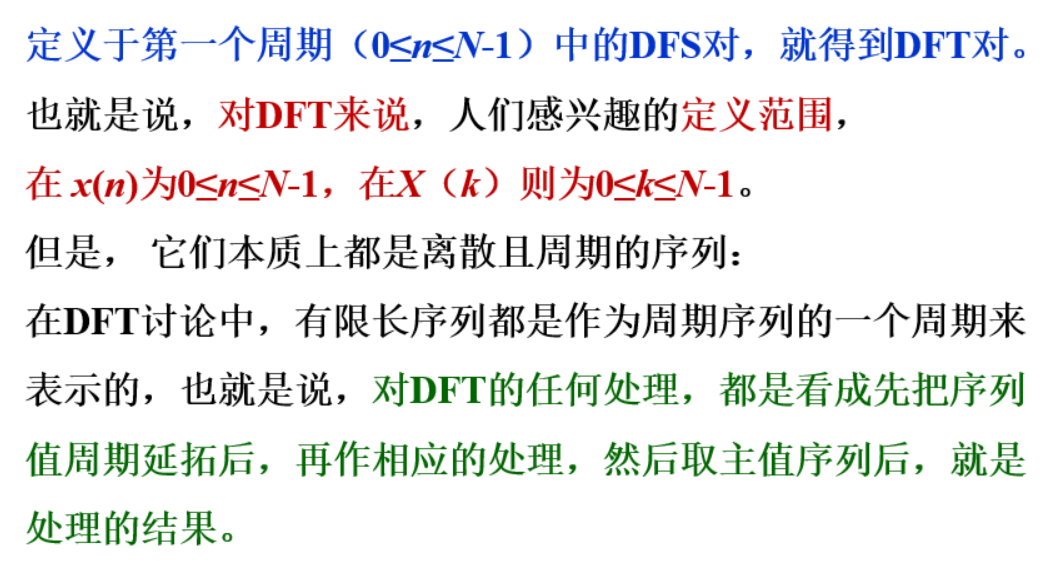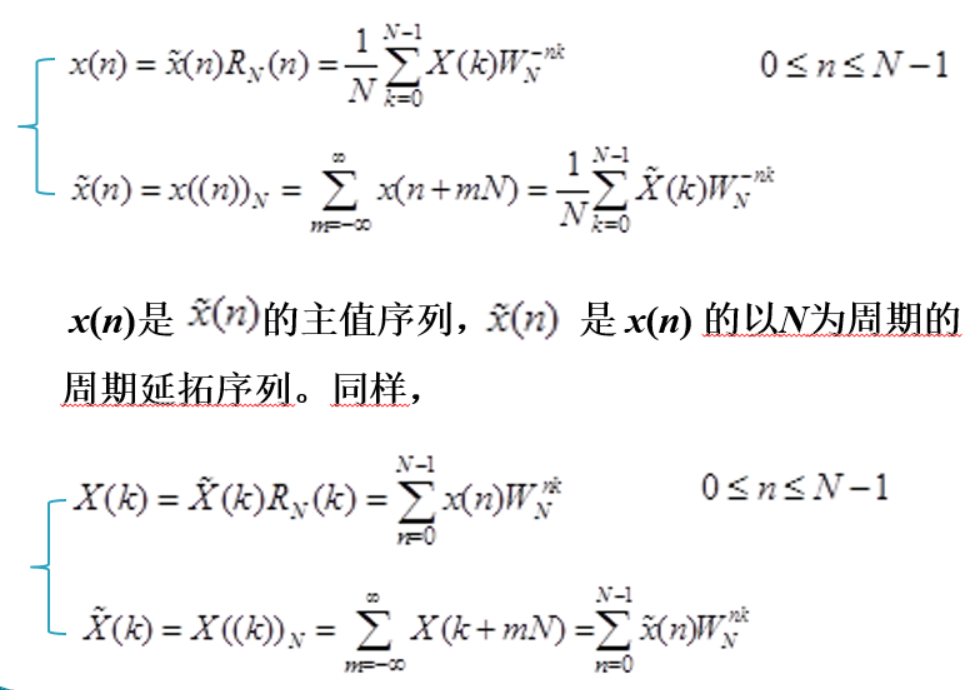5、DFT与DTFT、Z变换的关系 – 频域抽样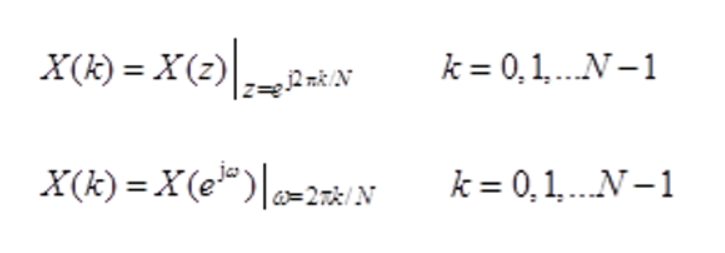6、离散傅里叶变换对x(n)与X(k)中各参量间的关系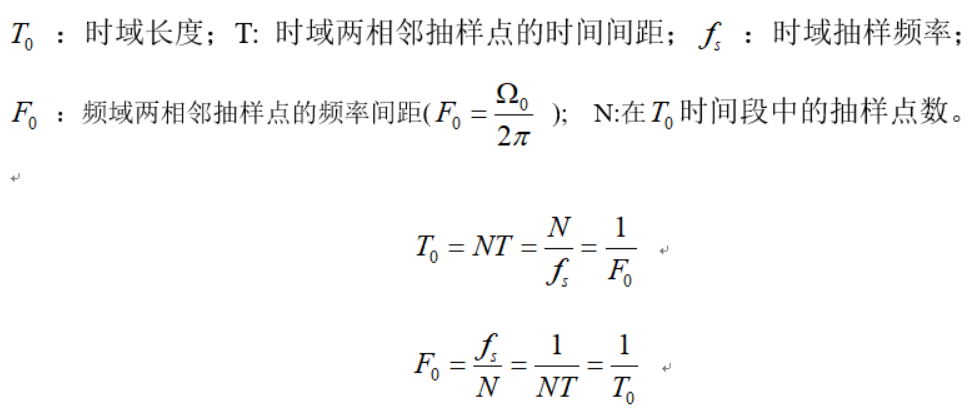（1） 时域相邻两抽样点的时间间距 等于抽样频率的倒数。
（2） 频域相邻两抽样点的频率间距 等于时域序列的时间长度的倒数。
（3）频域相邻两抽样点的频率间距也等于抽样频率 与抽样点数 N 之比值。
7、DFT 隐含的周期性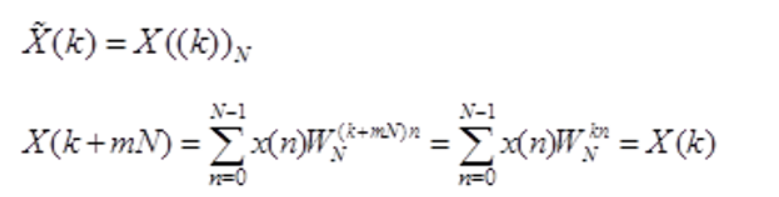二、DFT的主要性质
1、线性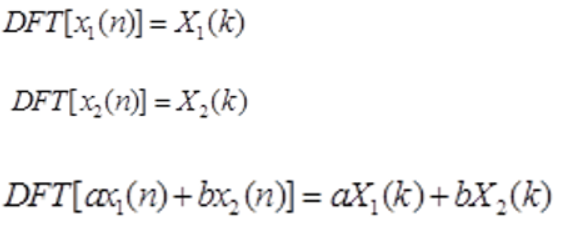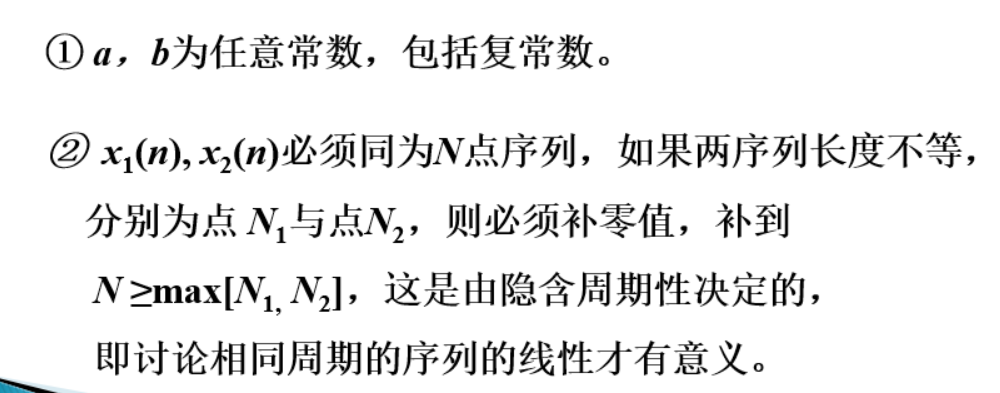2、圆周移位性质
2.1、圆周移位序列
一个有限长序列  $x(n)$ 的圆周位移是指用它的点数 N 为周期，将其延拓成周期序列  $\tilde x(n)$，将周期序列 $\tilde x(n)$ 加以位移，然后取主值区间（n 为 0~（N - 1））上的序列值。因此一个有限长序列 $x(n)$ 的m点圆周移位定义为：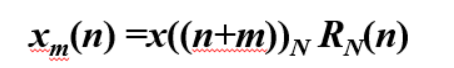上式中 $x{((n + m))_N}$ 表示  x(n) 的周期延拓序列 $\tilde x(n)$ 的 m 点线性移位
$x{((n + m))_N}{\rm{ = }}\tilde x(n + m)$
在作DFT运算时，N点有限长序列的m点移位，可以看成将x(n)以N为周期，延拓成周期序列 ，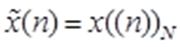将 x((n))N作m点线性移位后,再取主值区间中的序列，即得到x(n)的m点圆周移位序列 xm(n) 。
2.2、圆周移位性质
序列的圆周移位过程：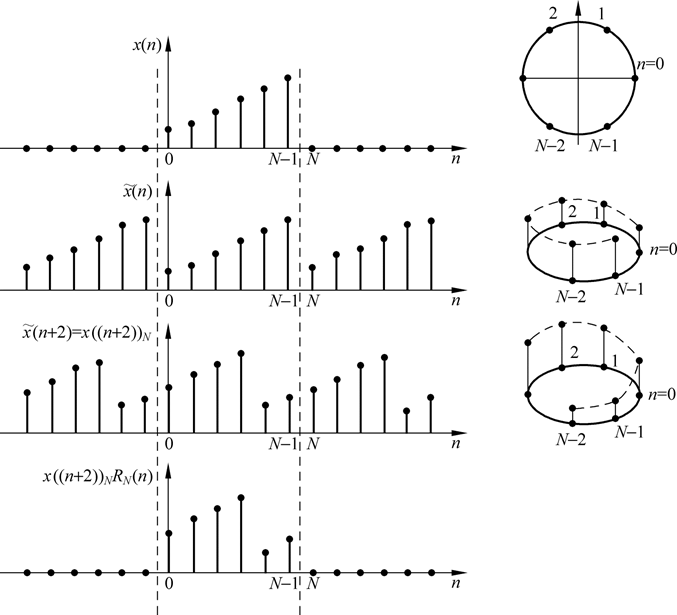上图中N=6。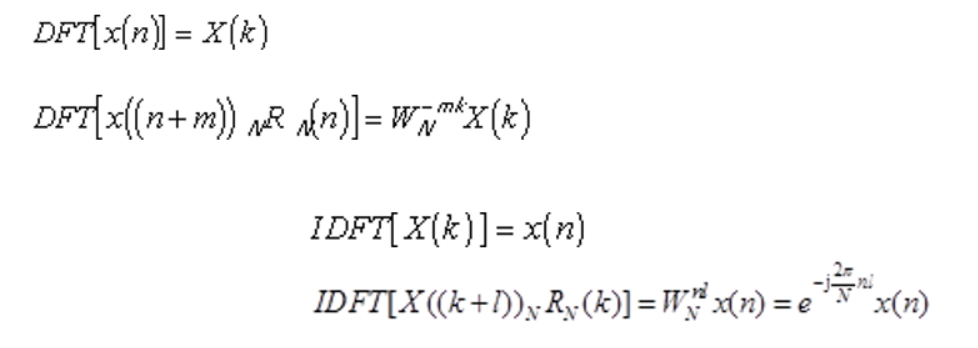调制特性 ~ 离散时域的调制（相乘）等效于离散频域的圆周移位。
2、圆周共轭对称性质
2.1、圆周对称中心
（未完待续！）


展开全文• 离散傅里叶变换（DFT）与相关性计算 文章目录离散傅里叶变换（DFT）与相关性计算基本定义时域的卷积对应频域的相乘循环平移的傅里叶变换相关与卷积通过傅里叶变换计算相关 本文主要介绍通过离散傅里叶变换的方法...卷积 fft
• DFT:(Discrete Fourier Transform)离散傅里叶变换是傅里叶变换在时域和频域上都呈离散的形式，将信号的时域采样变换为其DTFT的频域采样。在形式上，变换两端（时域和频域上）的序列是有限长的，而实际上这两组序列...FFT 一维FFT
• 离散傅里叶变换1.1 离散傅里叶变换与傅里叶变换1.2 离散傅里叶变换之后得到了什么1.3 离散傅里叶变换的共轭性2. 二维离散傅里叶变换2.1 二维离散傅里叶变换的实质2.2 二维离散傅里叶变换储存的复数的含义2.3 二维...
• 离散傅里叶变换（DFT） 定义 离散傅里叶变换（Discrete Fourier Transform，缩写为DFT），是傅里叶变换在时域和频域上都呈离散的形式，将信号的时域采样变换为其DFT的频域采样。 对于N点序列{X[n]}(0 <= n &...
• ## 离散傅里叶变换

千次阅读 2017-01-17 23:37:32
• DFT在零点 $\underline{\mathcal{F}}\underline{f}(0) = \displaystyle{ \sum_{n=0}^{N-1}\underline{f}[n]e^{-2\pi i\frac{n0}{N}} = \sum_{n=0}^{N-1}...还记得傅里叶变换在零点处也有类似的式子$\mathcal{F}...
• 离散傅里叶变换(DFT) 从前面我们已经知道，非周期连续函数傅里叶变换如下 F(ω)=∫−∞+∞f(t)e−iωtdt F(\omega)=\int ^{+\infty}_{-\infty}f(t)e^{-i\omega t}dt F(ω)=∫−∞+∞​f(t)e−iωtdt 单位冲激函数的...算法 卷积
• 废话不多说了，先看一下离散傅里叶变换的公式： ﻿公式1 看着如此简单的公式，其实暗藏玄机，往往我们忽略的都是最重要的细节。第一，这个公式针对的是离散周期信号，得到的频域也是离散周期频信号。这也是最普遍...
• 图像处理 二维离散傅里叶变换DFT matlab代码图像处理领域离散傅里叶变换的作用二维离散傅里叶变换二维离散傅里叶变换公式将二维的离散傅里叶变换进行转化将系数转化为矩阵形式注意，从矩阵的乘积i形式可以看出，原来...图像处理代码 matlab
• OpenCV C++离散傅里叶变换离散傅里叶变换程序说明代码运行效果 离散傅里叶变换 傅里叶变换是什么？一看就懂，写的超级棒！https://blog.csdn.net/m0_51233386/article/details/114764782 离散傅里叶变换（Discrete ...opencv 计算机视觉
• 应用：二维离散傅里叶变换是将图像从空间域转至频域，在图像增强、图像去噪、图像边缘检测、图像特征提取、图像压缩等等应用中都起着极其重要的作用。 **理论基础：**任意函数都可以表示成正弦函数的线性组合的形式...
• 通过数学方法自行推导了周期数列的傅里叶级数与有限数列的离散傅里叶变换数学 傅里叶
• 文章目录傅里叶级数与傅里叶变换离散傅里叶变换 傅里叶级数与傅里叶变换 这个学过信号与系统应该都挺了解的 傅里叶级数 傅里叶变换 离散傅里叶变换 DFT将一个采样函数从一个域转换到频域。 离散信号和周期信号最重要...
• 应用：二维离散傅里叶变换是将图像从空间域转至频域，在图像增强、图像去噪、图像边缘检测、图像特征提取、图像压缩等等应用中都起着极其重要的作用。理论基础：任意函数都可以表示成正弦函数的线性组合的形式。二维...
• 离散傅里叶变换 (DFT)，是傅里叶变换在时域和频域上的离散呈现形式，通俗的说就是将经过采样的有限长度时域离散采样序列变换为等长度的频域离散采样序列，通过对变换得到的频域采样序列进行适当的换算和处理，可以...matlab simulink fft idft 信号处理...